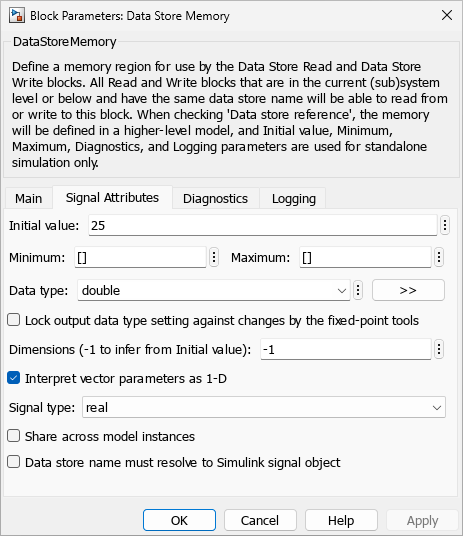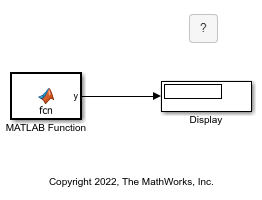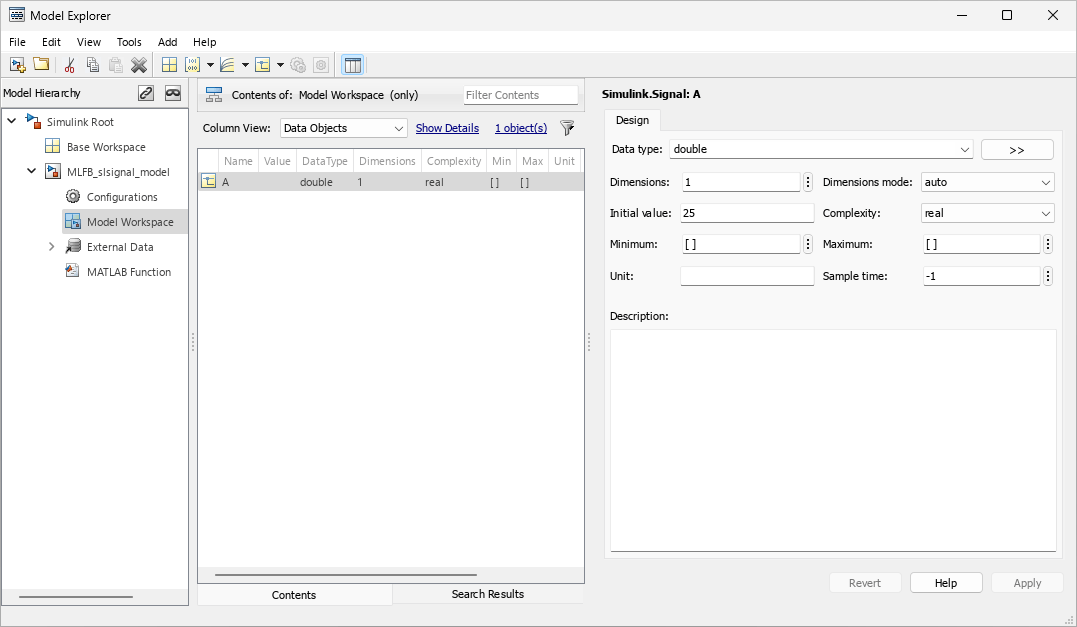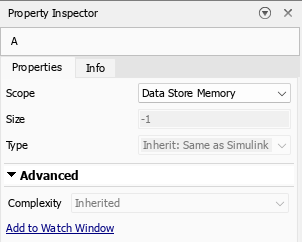## Use Global Data in MATLAB Function Blocks

You can reference globally defined data in MATLAB Function blocks by defining global variables in the function code. Use global variables if:

• You have multiple MATLAB® functions that use global variables and you want to call these functions from MATLAB Function blocks.

• You add a MATLAB Function block to an existing model that uses global data, and you want to avoid cluttering your model with additional inputs and outputs.

• You want to scope the visibility of data to parts of the model.

In Simulink®, you store global data using either Data Store Memory blocks or `Simulink.Signal` objects. For more information, see Local and Global Data Stores.

### Use Global Data from Data Store Memory Blocks

To define global data with a Data Store Memory block and use the data in a MATLAB Function block, or in code that this block calls:

1. Declare a global variable in your MATLAB Function block or in the code that the MATLAB Function block calls.

2. In the MATLAB Function block, set the Scope property of the global variable to `Data Store Memory`. For more information on how to define variables in MATLAB Function blocks, see Create and Define MATLAB Function Block Variables.

3. In the model, create a Data Store Memory block. Assign the Data store name parameter to the same name as the global variable.

4. In the Data Store Memory, set the Initial value, Data type, and Signal type parameters. The data type cannot be inherited, and the signal type must be real or complex.

Data Store Memory blocks scope the data to the model. You must add a Data Store Memory block to the model for each global data. Data Store Memory blocks do not support MATLAB value classes or variable-size data.

### Use Global Data from `Simulink.Signal` Objects

To define global data with a `Simulink.Signal` object and use the data in a MATLAB Function block or in the code that this block calls:

1. Declare a global variable in your MATLAB Function block, or in the code that the MATLAB Function block calls.

2. In the MATLAB Function block, set the Scope property of the global variable to `Data Store Memory`. For more information on how to define variables in MATLAB Function blocks, see Create and Define MATLAB Function Block Variables.

3. In the model workspace or base workspace, create a `Simulink.Signal` object. Assign the `Simulink.Signal` object to a variable with the same name as the global variable.

4. Set the `DataType`, `InitialValue`, and `Dimensions` properties of the `Simulink.Signal` object. The data type cannot be inherited, and the signal type must be real or complex.

You can scope `Simulink.Signal` objects to the model or base workspace. You can define the `Simulink.Signal` objects in the Model Explorer or load them from a MAT-file.

### Choose How to Store Global Data

How you store global data depends on the number and scope of your global variables. This table describes when to use Data Store Memory blocks or `Simulink.Signal` objects.

How You Use Global DataSolution
A single model that does not use a referenced model must define a small number of global variables.

Data Store Memory blocks.

A single model that does not use a referenced model must define a large number of global variables.

`Simulink.Signal` objects defined in the model workspace.

You share global data between multiple models, including referenced models.

`Simulink.Signal` objects defined in the base workspace.

### Retrieve Data From Data Store Memory Blocks

This example shows how a MATLAB Function block can use global data stored in a Data Store Memory block.View the Data Store Memory Block Parameters

Open the Data Store Memory block to view the parameters. On the Main tab, note that the Data store name parameter is `A`. Open the Signal Attributes tab. In this example, the Initial value parameter is `25`, the Data type parameter is `double`, and the signal type is real.To use global data in the MATLAB Function block, you cannot set these parameters to auto or inherited.

Inspect the MATLAB Function Block

Open the MATLAB Function block. The function code declares a global variable `A`, which matches the name of the Data Store Memory block Data store name parameter. The block adds `1` to `A` during each execution by using this code:

```function y = fcn global A; A = A+1; y = A; ```

Ensure that the variable `A` uses data store memory from the block:

1. In the Function tab, in the Prepare section, click Edit Data.

2. In the Symbols pane, select the variable `A`. The properties display in the Property Inspector.

3. Ensure the Scope property is `Data Store Memory`.Simulate the Model

Run the model. The block execution occurs at each major time step. The final output of the MATLAB Function block is `76`.

### Retrieve Data From `Simulink.Signal` Objects Example

This example shows how a MATLAB Function block can use the global data stored in a `Simulink.Signal` object.View the `Simulink.Signal` Object Properties

View the properties of the `Simulink.Signal` object:

1. Open the Model Explorer. In the Modeling tab, in the Design section, click Model Explorer.

2. In the left pane, expand the `MLFB_slsignal_model` and click Model Workspace. The middle pane displays the data in the model workspace.

3. Click the `Simulink.Signal` object `A`. In the right pane, the Model Explorer displays the properties of `A`. In this example, Data type is `double`, Dimensions is `1`, Initial value is `25`, and the complexity is real.To use global data in the MATLAB Function block, you cannot set these properties to auto or inherited.

Inspect the MATLAB Function Block

Open the MATLAB Function block. The function code declares a global variable `A`, which matches the name of the Data Store Memory block Data store name parameter. The block adds `1` to `A` during each execution by using this code:

```function y = fcn global A; A = A+1; y = A; ```

Ensure that the variable `A` uses data store memory from the object:

1. In the Function tab, in the Prepare section, click Edit Data.

2. In the Symbols pane, select the variable `A`. The properties display in the Property Inspector.

3. Ensure the Scope property is `Data Store Memory`.Simulate the Model

Run the model. The block execution occurs at each major time step. The final output of the MATLAB Function block is `76`.

### Using Data Store Diagnostics to Detect Memory Access Issues

If you use Data Store Memory blocks, you can avoid problems with data stores by configuring your model to provide run-time and compile-time diagnostics. The Configuration Parameters window and the Parameters window display diagnostics for the Data Store Memory block. For more information on using data store diagnostics, see Data Store Diagnostics.

Note

If you pass data store memory arrays to functions, optimizations such as ```A = foo(A)``` might result in the code generation software marking the entire contents of the array as read or written even though only some elements were accessed.